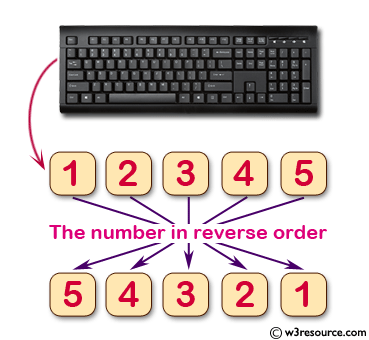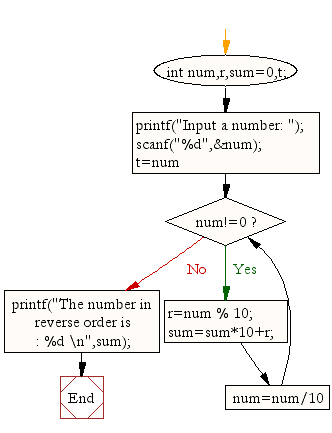﻿ C Program: Display the number in reverse order - w3resource# C Exercises: Display the number in reverse order

## C For Loop: Exercise-37 with Solution

Write a program in C to display the number in reverse order.

Pictorial Presentation:Sample Solution:

C Code:

``````#include <stdio.h>

void main(){
int num,r,sum=0,t;

printf("Input a number: ");
scanf("%d",&num);

for(t=num;num!=0;num=num/10){
r=num % 10;
sum=sum*10+r;
}
printf("The number in reverse order is : %d \n",sum);
}
```
```

Sample Output:

```Input a number: 12345
The number in reverse order is : 54321
```

Flowchart:C Programming Code Editor:

Improve this sample solution and post your code through Disqus.

What is the difficulty level of this exercise?

Test your Programming skills with w3resource's quiz.

﻿

## C Programming: Tips of the Day

Is there a way to specify how many characters of a string to print out using printf()?

The basic way is:

`printf ("Here are the first 8 chars: %.8s\n", "A string that is more than 8 chars");`

The other, often more useful, way is:

`printf ("Here are the first %d chars: %.*s\n", 8, 8, "A string that is more than 8 chars");`

Here, you specify the length as an int argument to printf(), which treats the '*' in the format as a request to get the length from an argument.

You can also use the notation:

```printf ("Here are the first 8 chars: %*.*s\n",
8, 8, "A string that is more than 8 chars");```

This is also analogous to the "%8.8s" notation, but again allows you to specify the minimum and maximum lengths at runtime - more realistically in a scenario like:

`printf("Data: %*.*s Other info: %d\n", minlen, maxlen, string, info);`

The POSIX specification for printf() defines these mechanism

Ref : https://bit.ly/3u32GyO